Finite Heat Release with Heat Transfer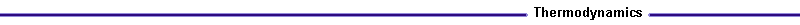Introduction

In spark ignition engines, the primary heat transfer mechanism from the cylinder gases to the wall is convection, with only 5% from radiation. Using a Newtonian model, the heat loss to the wall is given by:

Q = hA(Tg-Tw)
Where:
h = heat transfer coefficient
A = exposed combustion chamber surface area
Tg = temperature of the cylinder gas
Tw = cylinder wall temperature

Link to: Heat Transfer Coefficient Applet

Derivation of Pressure versus Crank Angle with the addition of Heat Transfer

When determining the heat release term,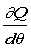, the heat loss to the walls has to be taken into account. The pressure over crank angle change now becomes:Where: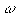= engine speed [rad/s]

The surface area of the cylinder can be determined from the slider-crank model.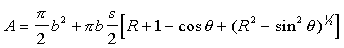The temperature of the gas, Tg, will be an average temperature. The temperature will be found from ideal gas relationships.Where:
M = molar mass of the fuel/air mixture
m = mass of the fuel/air mixture= universal gas constant

Using the relationship, r = V1/V1-Vd:Heat Transfer Coefficient Correlations

Two correlations developed by Annand and Woschni to determine the heat transfer coefficient will be discussed.  The first developed by Annand is the simpler of the two.

Annand developed the following correlation:Where:
hc      =    heat transfer coefficient
b      =    bore
k      =    thermal conductivity    =    0.15 W/mK
m      =    dynamic viscosity        =    7x105 kg/ms=    mean piston speed      =     2sw
a      =    varies from 0.35 to 0.8 for normal combustion
b      =    0.7

Woschni accounts for the increase in the gas velocity in the cylinder during combustion.  Annand assumed a constant characteristic gas velocity equal to the mean piston speed.  The average cylinder gas velocity, v, for a 4-stroke engine without swirl determined by Woschni is: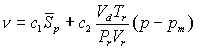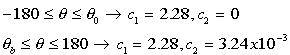Where:
Pmotor = motor pressure
Ta = ambient temperature, 298 K

Pa = atmospheric pressure, 1 atm

The motoring pressure is given by:The heat transfer coefficient given by Woschni is:A finite heat release calculation with the Woschni heat transfer model is given in the applet:  Finite Heat Release with Heat Transfer Applet. The applet can be used to compare the effect of adding cylinder heat transfer to the finite heat release model.